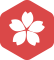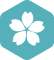### 正则匹配金额

.NET技术 > ASP.NET [问题点数：20分，结帖人ttg520]2005年9月 .NET技术大版内专家分月排行榜第三ttg520

js正则匹配验证价格、金额

_normalize(inValue) { if ( /^(([1-9][0-9]*)|((\.\d{0,2}|[1-9][0-9]*\.\d{0,2})))\$/.test(inValue)) { console.log('有效'); return inValue; } else { if (inValue.length === 1 &&

private static final String AMT_REGEX = "^(([1-9][0-9]{0,14})|({1})|((\\.\\d{1,2}|[1-9][0-9]{0,14}\\.\\d{1,2})))\$"; private static final String INPUT = "0.11"; public static void main(String...

js正则表达式校验金额

Java 金额正则表达式

Pattern pattern = Pattern.compile(“^((([1-9]{1}//d{0,9}))|({1}))((//.(//d){2}))?\$”); Matcher matcher = pattern.matcher(“3333333333.99”); System.out.println(matcher.matches());

&lt;input type="number" name="" id="num"&gt; &lt;script&gt; \$("#num").keyup(function(){ ...\$)|(^(0){1}\$)|(^[0-9]\.[0-9]{1,2}\$

js正则匹配金额(允许负数)

var pattern = new RegExp(/^([-+])?\d+(\.[0-9]{1,2})?\$/), str = '-1', str1 = '-1.1', str2 = '1.23'; console.log(pattern.test(str)); console.log(pattern.test(str1));...console.log(pattern.test(str2));

2019独角兽企业重金招聘Python工程师标准>>> ...

java 正则匹配数据中的金钱数字

Python结合正则匹配与分句的方式提取文本中的金额

MySQL正则匹配金额，前面可以有正负号，可以有千分符，可以有小数位

SELECT ('1299l' REGEXP '[^0-9.]'); 通过返回 1;不通过返回 0 -- 这个是MySQL支持语法： SELECT ('11,111,000.32' REGEXP '^(\\-|\\+){0,1}(...-- 查表 table_test 中 num 字段匹配通过的： SELECT num FROM table_t

HIVE正则匹配（深度应用）

php正则校验金额

js关于金额输入框正则匹配问题

java正则表达式验证金额

String reg_money = "...// 金额正则,可以没有小数，小数最多不超过两位 Pattern pattern = Pattern.compile(reg_money); Matcher matcher = pattern.matcher("5566.21"); boolean ismatch = ...

js 验证金额正则表达式

1，整数部分最多是三位数字 2，小数点后最多两位 例如：12.22 123.00 123.9 123.33 123 12

php 正则金额验证

\$money_reg = '/^[1-9]\d*|^[1-9]\d*.\d+[1-9]\$/';if(!preg_match(\$money_reg, \$money)){ \$this->ajaxError('金额格式错误');} 转载于:https://www.cnblogs.com/phpwyl/p/10178060.html

input数字正则控制（一般用于金额限制）

php 正则匹配字符串中的所有数字

php 正则匹配字符串中的所有数字 function isHaveKC(\$str){ preg_match_all('/\d+/',\$str,\$arr); print_r(\$arr); } echo isHaveKC("第1-4周 第14-18周");  执行结果： Array (  =&gt; ...

JS正则匹配充值时的 0.01的整数倍

var reg = /^0\.([1-9]|\d[1-9])\$|^[1-9]\d{0,8}\.\d{0,2}\$|^[1-9]\d{0,8}\$/; var amount = 0.02; /^0\.([1-9]|\d[1-9])\$|^[1-9]\d{0,8}\.\d{1,2}\$|^[1-9]\d{0,8}\$/; reg.test(amount);

var a = 222122122.6754; var b = a.toFixed(2).replace(/(\d)(?=(\d{3})+\.)/g, '\$1,');//使用正则替换，每隔三个数加一个','

【JavaScript/jQuery】JavaScript 正则 验证金额（小数位不超过2位）

Python正则匹配数字和小数

1. 正整数或有1/2位小数： var reg1 = new RegExp('(^[1-9][0-9]*)+(.[0-9]{1,2})?\$');  2. 0~1之间的小数： var reg2 = new RegExp('^0[.][0-9]{1,2}\$');  3. 0 ： var reg3 = new RegE

JS正则匹配验证金额，可为整数，一位小数或两位小数

input class="text check_num" type="text" name="money" value="{\$money}" rel="{\$money}" placeholder="请输入金额" /> /** * 检测金额，键盘松开时触发的事件 keyup */ \$(".check_num").on("keyup",...